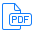# Trapezoidal Generalization of Lustre with Uninterpreted Functions## D. Greve, J. Backes

### Technical Report. 2017

Model-based fuzzing is a fuzzing technique that employs a mathematical model of system behavior to guide the fuzzing process and explore behaviors that would otherwise be difficult to reach by chance. Whereas traditional fuzzing frameworks generate tests randomly, a model-based framework can deduce tests from a behavioral model using a constraint solver. We are developing FuzzM, a model-based fuzzing framework that employs Lustre as a modeling language and leverages the JKind model checker as a constraint solver. Because the state space being explored by the fuzzer is often large, the rapid generation of test vectors is crucial. The need to generate tests quickly, however, is antithetical to the use of a constraint solver. Our solution to this problem is to use JKind to generate an initial solution and then to perform trapezoidal generalization of the solution relative to the Lustre specification. Test generation then consists of rapid, repeated, randomized sampling of trapezoidal generalization spaces. Trapezoidal generalizations are ordered, hierarchical conjunctions of linear constraints. They are more expressive than simple intervals but are more efficient to manipulate and easier to sample than generic polytopes. In this paper we describe an approach to the trapezoidal generalizations of JKind counterexamples relative to Lustre models with integer division, remainder, and uninterpreted functions. We demonstrate our approach on a Lustre specification that recognizes permutations of complete integer sequences by generalizing a counterexample sequence satisfying the permutation property to produce a family of permuted sequences that can be rapidly sampled.